# Problem of the Month (October 1999)

This month we play with arrangements of squares of side 1, 2, 3, . . . n in the plane. We want all these squares to be non-overlapping, unrotated, and have vertices with integer corrdinates.

### Question #1:

If these squares are "stacked" on a flat surface, what is the longest flat surface that rests on some of these squares? Squares must be fully supported below. For example, f(5)=8: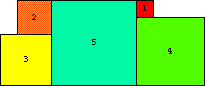Let f(n) be the longest flat surface obtainable from the squares 1, 2, 3, . . . n. Can you find good bounds for f(n)? What can you prove about f(n)? Does f(n)/n → ∞ as n→ ∞?

### Question #2:

For which n does there exist an edge-connected arrangement of these n squares so that the area covered has some sort of symmetry?

### Question #3:

What is the smallest square that these n squares will fit into? This is an old question, dating back to Martin Gardner's column in Scientific American some 30 years ago. This sequence starts 1, 3, 5, 7, 9, 11, 13, 15, 18, 21, 24, 27, 30, 33, 36, 39, 43, 47, 51, 54, 58, 63, 67, 71, . . . and is A005842 of the Encyclopedia of Integer Sequences. Can anyone calculate the next term, involving squares of sides 1, 2, 3, . . . 25?

### Question #1:

Joseph DeVincentis found most of the best bounds on f(n) for n≤30. He also showed that f(99) ≥ 892. He made the following conjecture, which would imply that f(n)/n → ∞ as n→ ∞. This is based on a flat surface at height roughly 2n.

Conjecture: The optimal solutions for large n have two layers and f(n) ≈ (1+4+9+ . . . +n2) / 2n ≈ n2/6.

Trevor Green showed f(9999) ≥ 165448. He also proved that f(n)/n → ∞ by showing

Theorem: Let G(n)=log(√3(2n–6)/(1+√3))/ log(2+√3). Then f(n) ≥ n (G(n)–2)/2.

Here are the best known lower bounds for f(n):

## Length of Longest Flat Surface

nf(n)PictureAuthor
11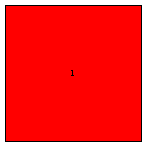Trivial
22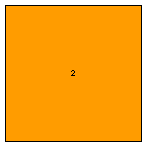Trivial
34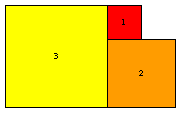Trivial
45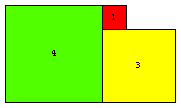Erich Friedman
58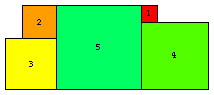Erich Friedman
69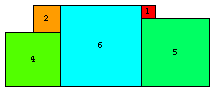Erich Friedman
712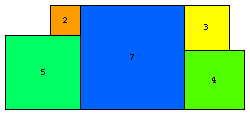Erich Friedman
813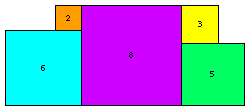Erich Friedman
918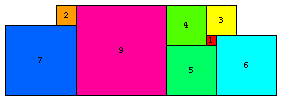Erich Friedman
1020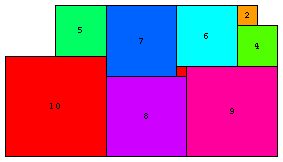Joe DeVincentis
1124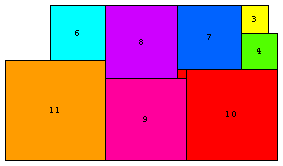Joe DeVincentis
1229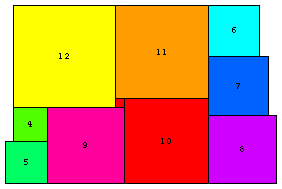Joe DeVincentis
1332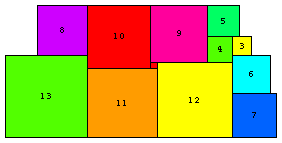Joe DeVincentis
1439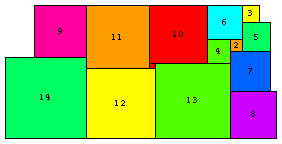Joe DeVincentis
1547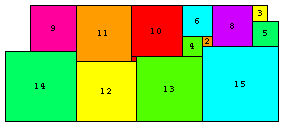Joe DeVincentis
1651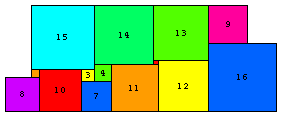Joe DeVincentis
1757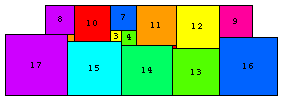Joe DeVincentis
1859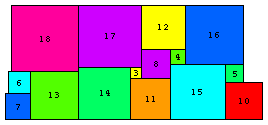Joe DeVincentis
1964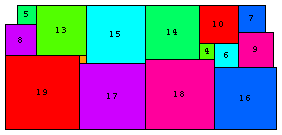Joe DeVincentis
2081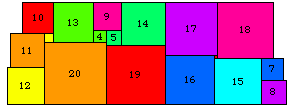Erich Friedman
2181Erich Friedman
2284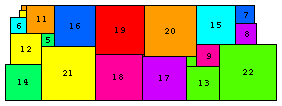Joe DeVincentis
2387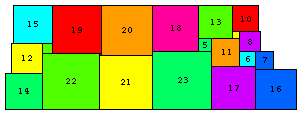Joe DeVincentis
2495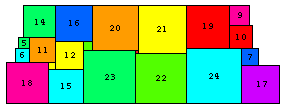Joe DeVincentis
25107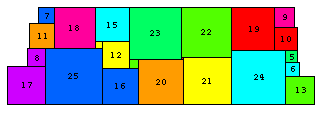Joe DeVincentis
26118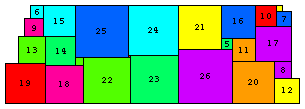Joe DeVincentis
27130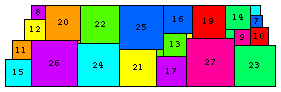Joe DeVincentis
28130Joe DeVincentis
29135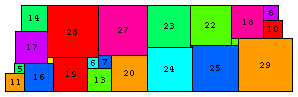Joe DeVincentis
30147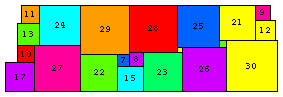Joe DeVincentis

### Question #2:

There were no responses, except to note that for n=1, 1 square is symmetric, and that there is a solution if the squares don't have to placed with lattice points as corners.

### Question #3:

There were no responses, though there was progress on this problem in 2004, 2009, and 2010. Here are the best known packings: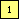s(1)=1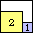s(2)=3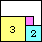s(3)=5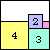s(4)=7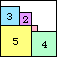s(5)=9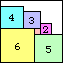s(6)=11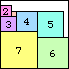s(7)=13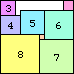s(8)=15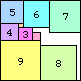s(9)=18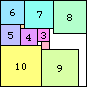s(10)=21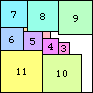s(11)=24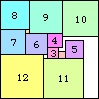s(12)=27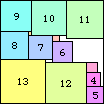s(13)=30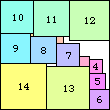s(14)=33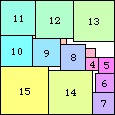s(15)=36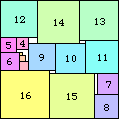s(16)=39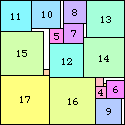s(17)=43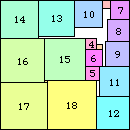s(18)=47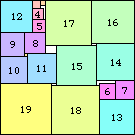s(19)=50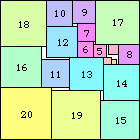s(20)=54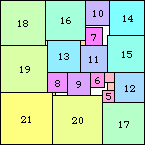s(21)=58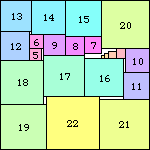s(22)=62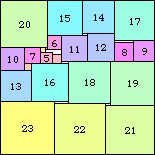s(23)=66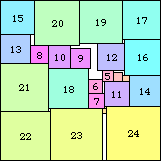s(24)=71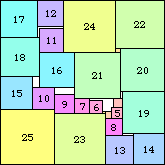s(25)=75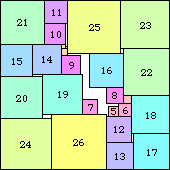s(26)=80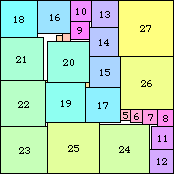s(27)=84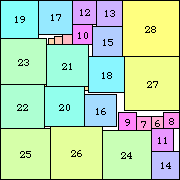s(28)≤89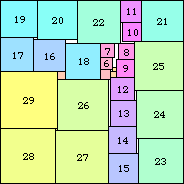s(29)=93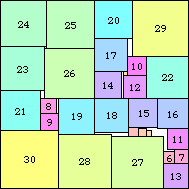s(30)=98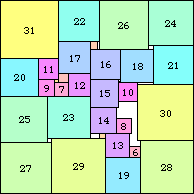s(31)=103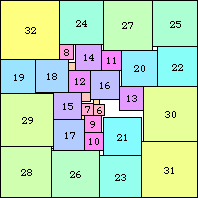s(32)=108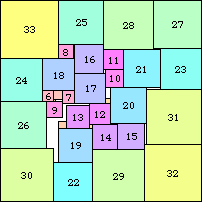s(33)=113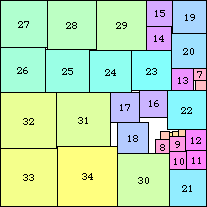s(34)=118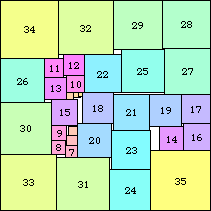s(35)=123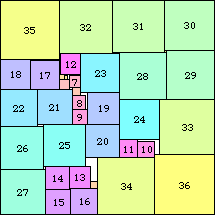s(36)=128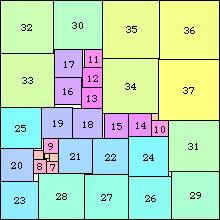s(37)=133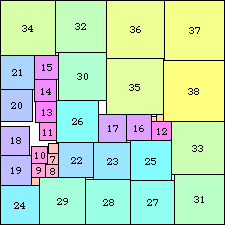s(38)≤139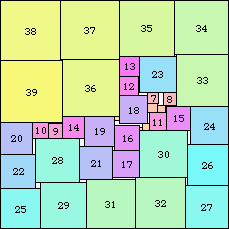s(39)=144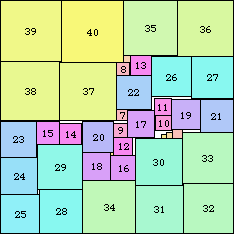s(40)≤150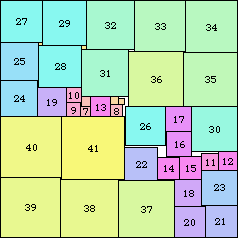s(41)=155

If you can extend any of these results, please e-mail me. Click here to go back to Math Magic. Last updated 10/10/10.